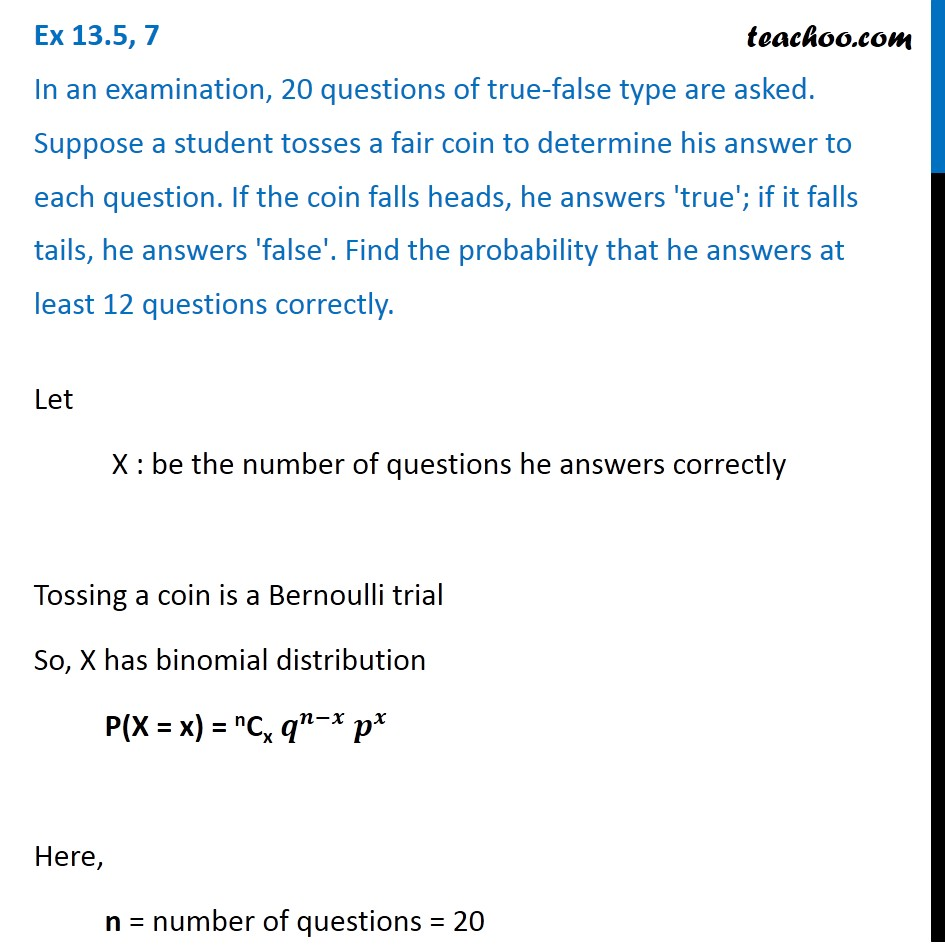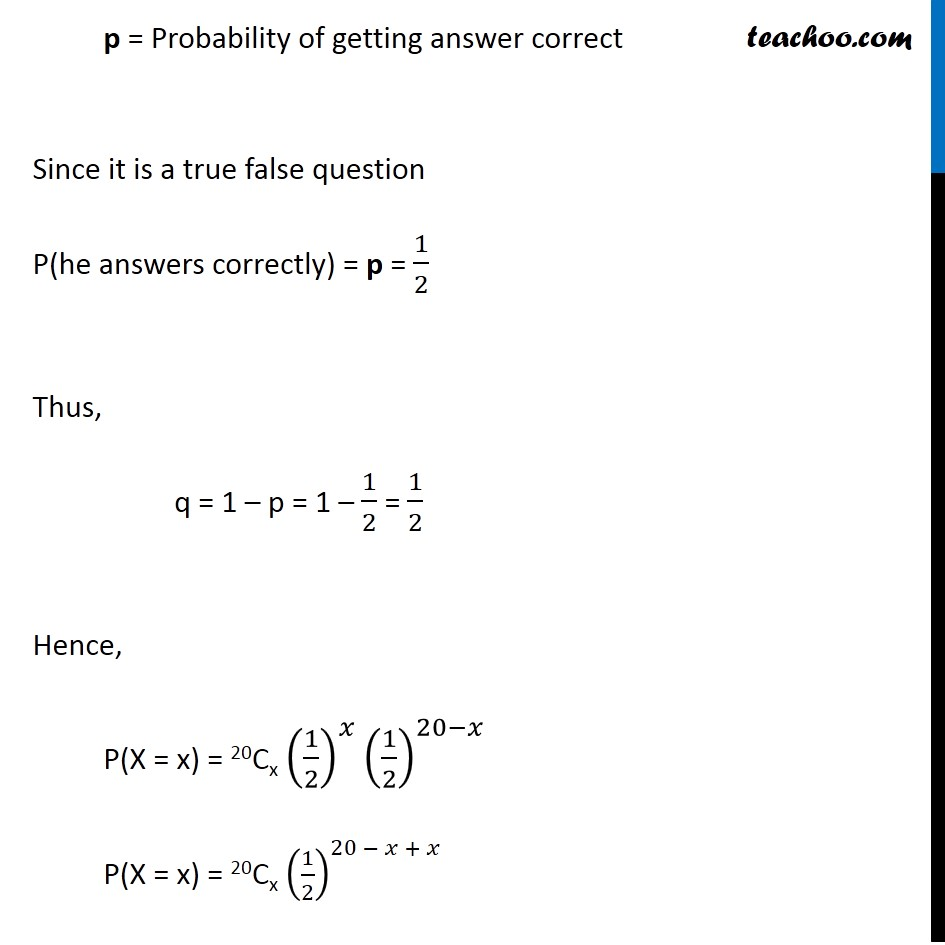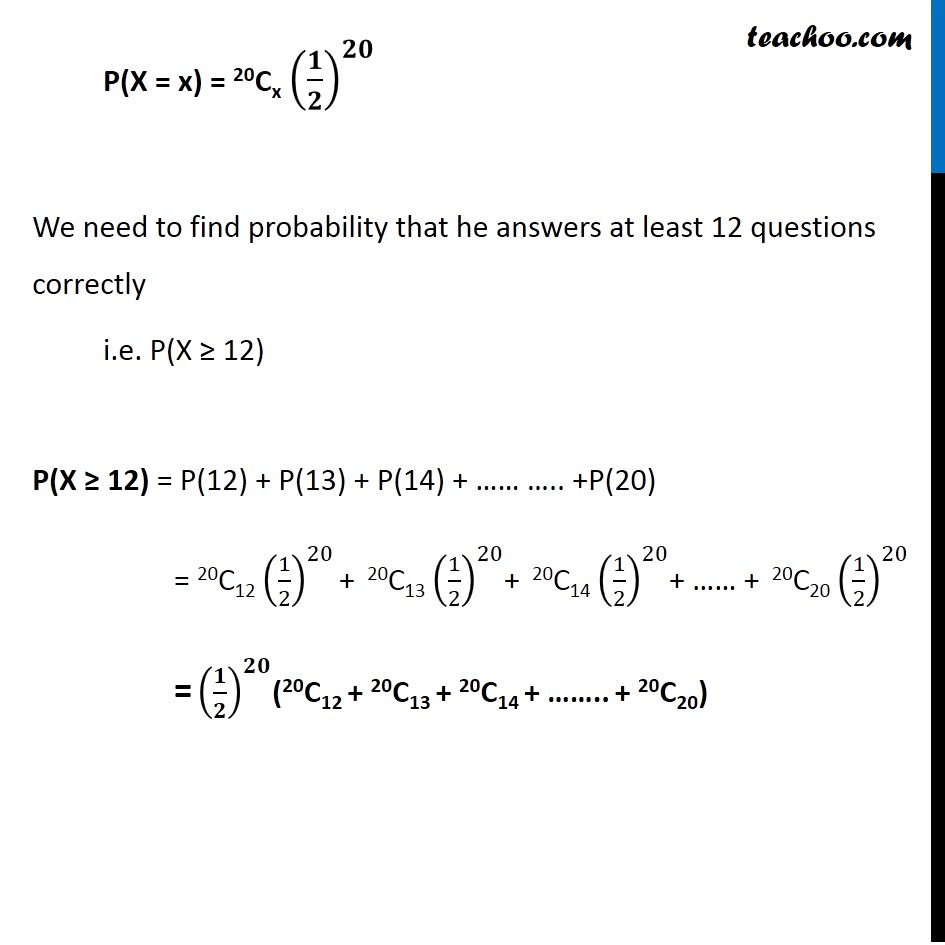Bernoulli Trial

Chapter 13 Class 12 Probability
Serial order wiseLearn in your speed, with individual attention - Teachoo Maths 1-on-1 Class

### Transcript

Question 7 In an examination, 20 questions of true-false type are asked. Suppose a student tosses a fair coin to determine his answer to each question. If the coin falls heads, he answers 'true'; if it falls tails, he answers 'false'. Find the probability that he answers at least 12 questions correctly.Let X : be the number of questions he answers correctly Tossing a coin is a Bernoulli trial So, X has binomial distribution P(X = x) = nCx 𝒒^(𝒏−𝒙) 𝒑^𝒙 Here, n = number of questions = 20 p = Probability of getting answer correct Since it is a true false question P(he answers correctly) = p = 1/2 Thus, q = 1 – p = 1 – 1/2 = 1/2 Hence, P(X = x) = 20Cx (1/2)^𝑥 (1/2)^(20−𝑥) P(X = x) = 20Cx (1/2)^(20 − 𝑥 + 𝑥) P(X = x) = 20Cx (𝟏/𝟐)^𝟐𝟎 We need to find probability that he answers at least 12 questions correctly i.e. P(X ≥ 12) P(X ≥ 12) = P(12) + P(13) + P(14) + …… ….. +P(20) = 20C12 (1/2)^20 + 20C13 (1/2)^20+ 20C14 (1/2)^20+ …… + 20C20 (1/2)^20 = (𝟏/𝟐)^𝟐𝟎(20C12 + 20C13 + 20C14 + …….. + 20C20)